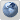FORUM CLOSED, PLEASE REGISTER AT FORUM.SIO2INTERACTIVE.COM
Would you like to react to this message? Create an account in a few clicks or log in to continue.

# calculate an angle rotation from a direction vector

2 posters##calculate an angle rotation from a direction vector

I have a vector representing a direction and I want my character to 'face' that direction. I know how to rotate the character on its z-axis with sio2 using SIOTransform 'rot' vector, but I need an angle for that (0->360 or -180->180 i think). I think i only need the X and Y values from the direction vector since its only rotating around one axis, so that would be a simple 2D operation. I'm thinking of a function that would take 2 vector arguments and return the angle between those 2 vectors.

What's the best way to do this in SIO2? Definitely a beginner question. Any help is appreciated.

geekschmoe

Posts : 14
Join date : 2009-06-22

##Re: calculate an angle rotation from a direction vector

OK so I figured it out. Simple 2D math (but theres no such thing as 'simple' math to me). On the offshoot that someone could be as beginner as me, here's how I calculated it.

Code:
`player->SIO2transform->rot->z = atan2(directionVector->y, directionVector->x) * 180 / M_PI; `

geekschmoe

Posts : 14
Join date : 2009-06-22

##Re: calculate an angle rotation from a direction vector

Hummm maybe more safe to do this:

Code:
`   float a = asinf( _v->x / hypotf( _v->x, _v->y ) ) * SIO2_RAD_TO_DEG;   if( _v->y < 0.0f )   { a += 180.0f; }   else   { a = 360.0f - a; }      return a;`sio2interactive

Posts : 1526
Join date : 2008-08-26
Age : 43
Location : Shanghai##Re: calculate an angle rotation from a direction vector